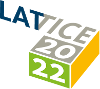#The 39th International Symposium on Lattice Field Theory (Lattice 2022)

Aug 8 – 13, 2022
Hörsaalzentrum Poppelsdorf
Europe/Berlin timezone

## QCD phase diagram in a magnetic background

Aug 12, 2022, 2:30 PM
20m
CP1-HSZ/0.011 (CP1-HSZ) - HS1 (CP1-HSZ)

### CP1-HSZ/0.011 (CP1-HSZ) - HS1

#### CP1-HSZ

450
Show room on map
Oral Presentation QCD at Non-zero Temperature

### Speaker

Lorenzo Maio (University of Pisa and INFN section of Pisa)

### Description

We discuss the QCD phase diagram in the presence of a strong magnetic background field. We provide numerical evidence, based on lattice simulations of QCD with 2+1 flavours and physical quark masses, for a crossover transition at $eB = 4$ GeV$^2$ (with a pseudo-critical temperature $T_c = (98±3)$ MeV) and for a first order phase transition at $eB = 9$ GeV$^2$ (where the measured critical temperature is $T_c = (63±5)$ MeV). We conclude that the critical endpoint is located at $eB=eB_{CEP}$ and $T=T_{CEP}$, where $4$ GeV\$^2

### Primary authors

Alfredo Stanzione (SISSA) Francesco Sanfilippo Lorenzo Maio (University of Pisa and INFN section of Pisa) Massimo D'Elia (Pisa U. and INFN Pisa)